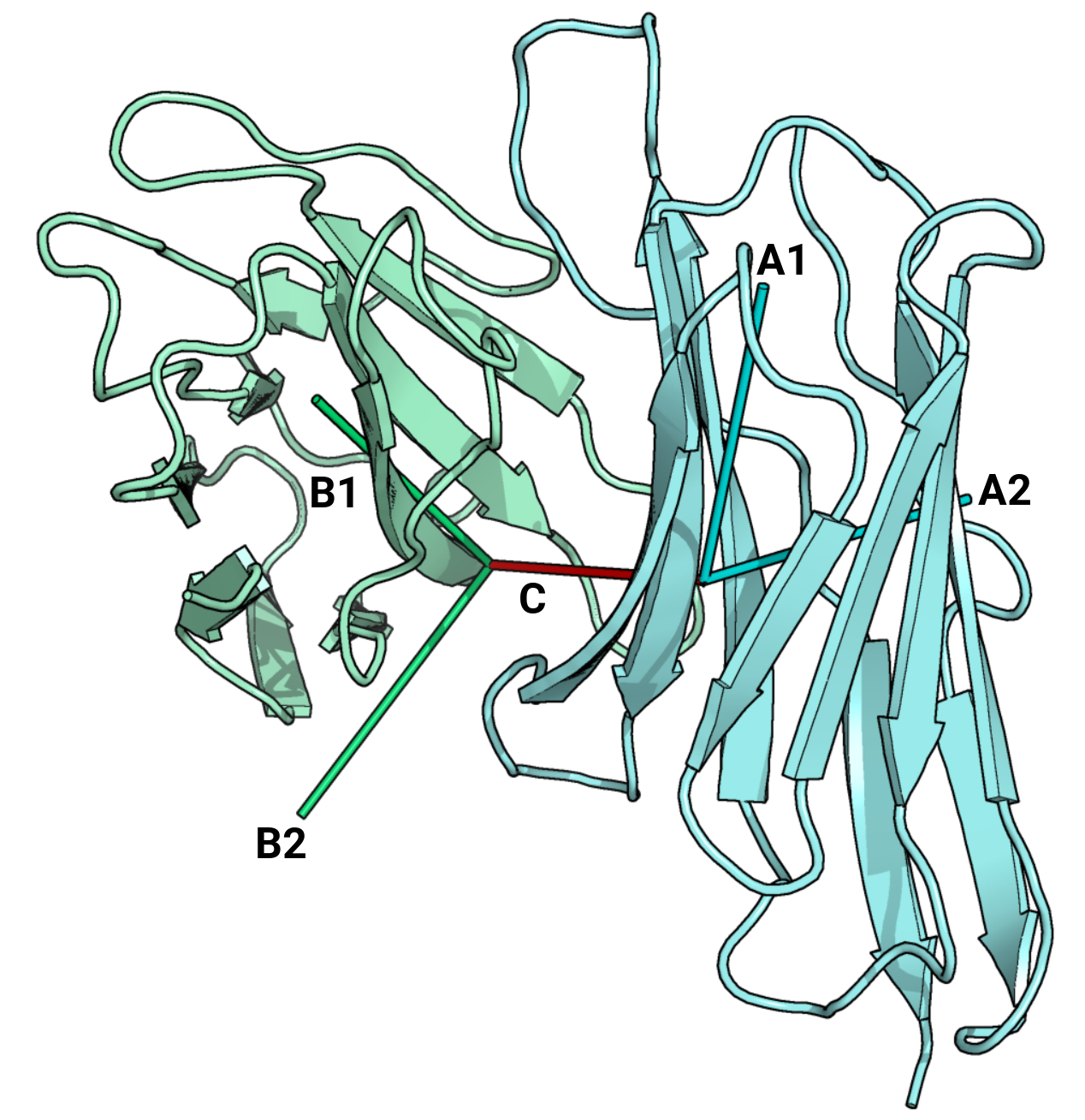# Orientation search

Search for TCRs based on the relative orientation of their variable domains.

The relative orientation between the variable domains (Vβ and Vα) can be represented by six metrics, known as the TRangles. These values act as a quantitative description of the TCR's antigen-binding site. These values are similar to the ABangle parameters that are used for antibodies. To calculate the similarity in orientations, we calculate the ABangle distance of the six ABangle/TRangle parameters between two TCRs or an antibody and a TCR. If the distance is less than 10, two structures are considered similar.BA the torsion angle between B1 and A1 BC1 the bend angle between B1 and C BC2 the bend angle between B2 and C AC1 the bend angle between A1 and C AC2 the bend angle between A2 and C dc the length of C
1. Search for TCR structures by selecting each TRangle.
2. Search for a specific TCR structure or find similar orientations.